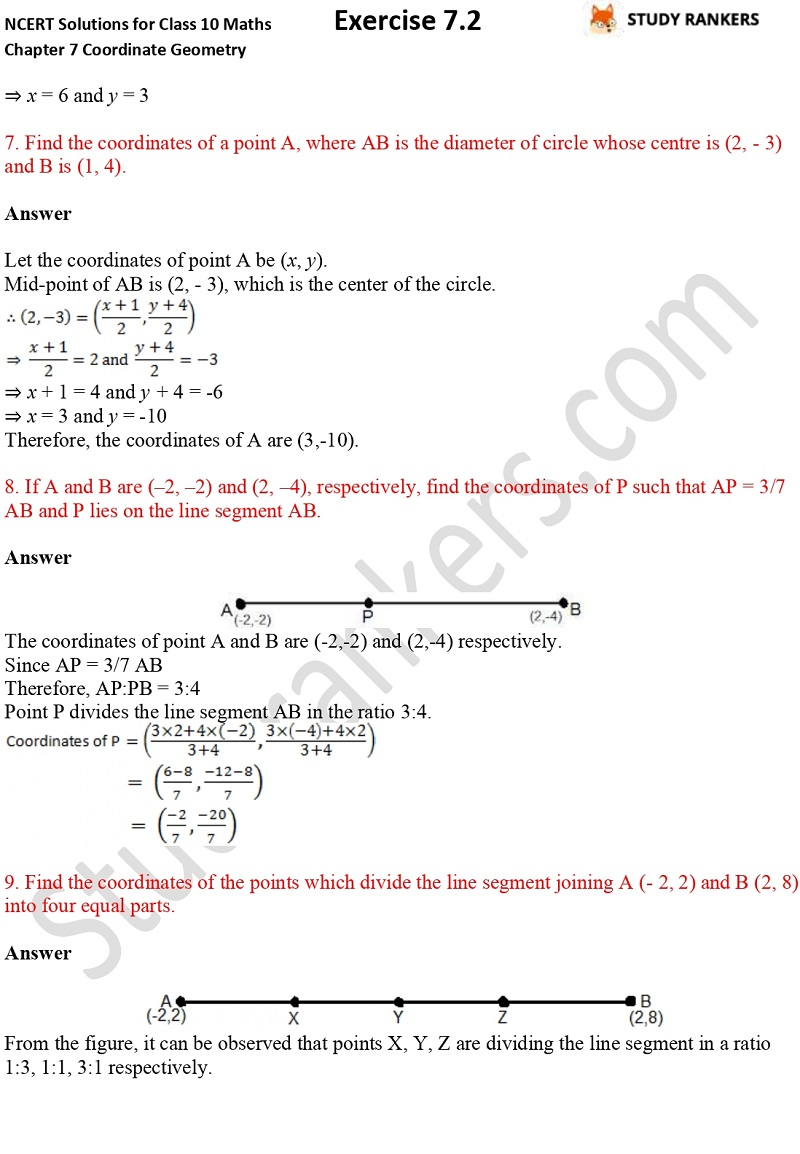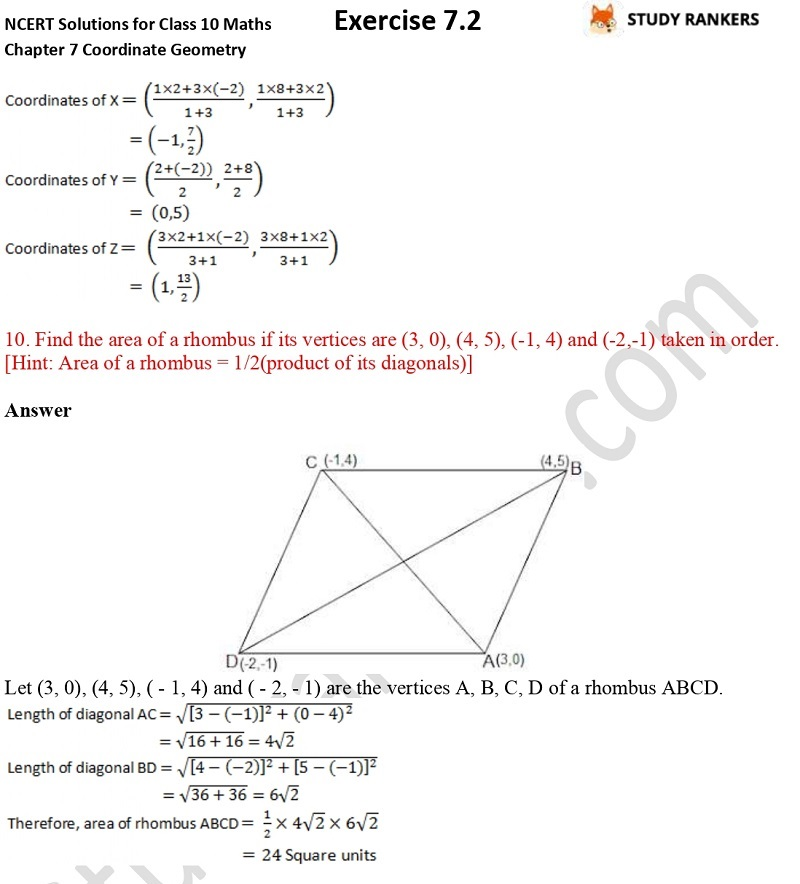## NCERT Solutions for Class 10 Maths Chapter 7 Coordinate Geometry Exercise 7.2

In this page, you will find Chapter 7 Coordinate Geometry Exercise 7.2 Class 10 Maths NCERT Solutions which will help you in completing your homework on time. These NCERT Solutions are prepared by Studyrankers experts who have taken care to minimize the errors and provide step by step solutions. These Maths Class 10 Solutions are updated as per the latest NCERT Textbooks 2019-20. You can take help from these while solving any difficult questions also match your answers with them.

The exercise contains total 10 questions such as find the coordinates of the point which divides the join of (- 1, 7) and (4, - 3) in the ratio 2:3, If (1, 2), (4, y), (x, 6) and (3, 5) are the vertices of a parallelogram taken in order, find x and y. etc.